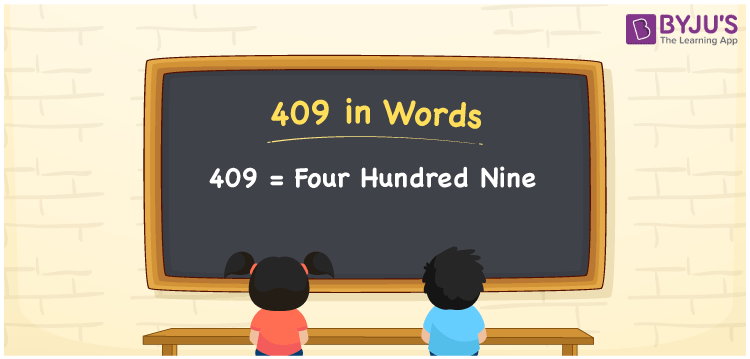# 409 in Words

409 in words is written as Four hundred nine. In both the International System of Numerals and the Indian System of Numerals, 409 is written as Four hundred nine. The number 409 is a Cardinal Number as it represents some quantity. For example, “there are 409 chairs in the room”.

 409 in Words Four hundred nine Four hundred nine in Number 409

## 409 in English Words

We write 409 in English Words using the letters of the English alphabet. Therefore, we read 409 in English as “Four hundred nine.”## How to Write 409 in Words?

To write 409 in words, we shall use the place value chart. In the place value chart, write 4 in the hundreds, 0 in the tens, and 9 in the ones, respectively. Now let us make a place value chart to write the number 409 in words.

 Hundreds Tens Ones 4 0 9

Thus, we can write the expanded form as

4 × Hundred + 0 × Ten + 9 × One

= 4 × 100 + 0 × 10 + 9 × 1

= 400 + 0 + 9

= 409

= Four hundred nine.

409 is a natural number, the successor of 408 and the predecessor of 410.

409 in words – Four hundred nine

• Is 409 an odd number? – Yes
• Is 409 an even number? – No
• Is 409 a perfect square number? – No
• Is 409 a perfect cube number? – No
• Is 409 a prime number? – Yes
• Is 409 a composite number? – No

## Frequently Asked Questions on 409 in Words

Q1

### How to write 409 in words?

409 in words is written as Four hundred nine.
Q2

### How to write 409 in the International and Indian System of Numerals?

In both, the system of numerals, 409 in words, is written as Four hundred nine.
Q3

### What is the preceding number of 409?

The number that precedes 409 is 408.